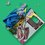# A fun function involving primes

$\displaystyle \large H(x) = \sum_{p \, | \, x} \left(p*\log_p({\text{gcf}(x,p^{\lfloor \log_p x \rfloor})})\right)$ The idea for this function was adding together the largest $np$ such that $p^n \mid x$ and $p^{n+1}\nmid x$ for a given $x$ and all prime $p\le\sqrt{x}$. For example, $H(28)=11$ since $28=7*2^2$.

Here's a list of the first 30 $H(x)$, starting from $x=2$: $2,3,4,5,5,7,6,6,7 ,11,7 ,13,9 ,8 ,8 ,17,8 ,19,9 ,10,13,23,9 ,10,15,9 ,11,29,10,31,\dots$

I'm interested in learning more about this function. To start things off, here's some identities I've already found: $H(p_n)=p_n$ $H(ab)=H(a)+H(b)$ $H(x^b)=b*H(x)$ $H(x!)=\sum_{k=0}^x H(k)=\sum_{k=2}^x H(k)$Note by William Crabbe
1 year, 9 months ago

This discussion board is a place to discuss our Daily Challenges and the math and science related to those challenges. Explanations are more than just a solution — they should explain the steps and thinking strategies that you used to obtain the solution. Comments should further the discussion of math and science.

When posting on Brilliant:

• Use the emojis to react to an explanation, whether you're congratulating a job well done , or just really confused .
• Ask specific questions about the challenge or the steps in somebody's explanation. Well-posed questions can add a lot to the discussion, but posting "I don't understand!" doesn't help anyone.
• Try to contribute something new to the discussion, whether it is an extension, generalization or other idea related to the challenge.

MarkdownAppears as
*italics* or _italics_ italics
**bold** or __bold__ bold
- bulleted- list
• bulleted
• list
1. numbered2. list
1. numbered
2. list
Note: you must add a full line of space before and after lists for them to show up correctly
paragraph 1paragraph 2

paragraph 1

paragraph 2

[example link](https://brilliant.org)example link
> This is a quote
This is a quote
    # I indented these lines
# 4 spaces, and now they show
# up as a code block.

print "hello world"
# I indented these lines
# 4 spaces, and now they show
# up as a code block.

print "hello world"
MathAppears as
Remember to wrap math in $$ ... $$ or $ ... $ to ensure proper formatting.
2 \times 3 $2 \times 3$
2^{34} $2^{34}$
a_{i-1} $a_{i-1}$
\frac{2}{3} $\frac{2}{3}$
\sqrt{2} $\sqrt{2}$
\sum_{i=1}^3 $\sum_{i=1}^3$
\sin \theta $\sin \theta$
\boxed{123} $\boxed{123}$

Sort by:

I found some decent upper and lower bounds for this: $2k \le H(n) \le \frac{n}{2^{k-1}} + 2(k-1)$

Where $k$ is the number of prime factors of $n$.

- 1 year, 3 months ago

In number theory there is a function $\log_{p}(x)=$ largest $n$ such that $p^{n}\mid x$. Or in algebra the $p$-adic evaluation delivers this $\nu_{p}(x)=n$. In any case, we can do away with the multiple layers of superfluous terms in your function and write it simply as

$H(x):=\sum_{p\in\mathbb{P}}p\log_{p}(x)$

Noting that $\log_{p}(0)$ should be defined as $\infty$, we have $H(0)=\infty$, not $0$(!!). I don’t know why you then say all prime $\leq\sqrt{x}$ that makes no sense. Then $H(p)$ would be $0$ for all $p\in\mathbb{P}$.

Anyhow. Of interest would be, if you can extend $H$ to $\mathbb{Q}$. Extending to $\mathbb{Z}$ is easy (just set $H(-n)=-H(n)$ or $H(-n)=H(n)$ however you like. Ideally, due to property 2, we would like to have $H(x/y)=H(x)-H(y)$. It’s easy to see that this is in fact well defined, provided we choose $H(-n)=H(n)$ above $\ldots$ and provided you set $H(0)=\infty$, otherwise you will run into problems.

Now set $|x|:=\exp(-H(x))$ for all $x\in\mathbb{Q}$. Then $|x|=0$ iff $x=0$ and $|\cdot|$ is multiplicative. The operation is however not a norm, since $|5+-3|=|2|=\exp(-2)>\exp(-5)+\exp(-3)=|5|+|-3|$.

- 1 year, 8 months ago

Another note: If we define a function $P_n(x)$ as the highest power of $n$ that divides $x$, we can express this function as $\sum_{n=1}^{\pi(\sqrt{x})} p_n P_{p_n}(x)$ where $\pi(x)$ is the prime counting function.

- 1 year, 9 months ago

how do we write an interger

- 1 year, 9 months ago

You don't mean all prime $p \le \sqrt{x},$ you mean all $p \le x,$ right?

The first two properties determine your function. If you look at $h(x) = 2^{H(x)},$ then $h$ is completely multiplicative.

There are some good links here. Apparently your function is sometimes called sopfr, for "sum of prime factors with repetition."

- 1 year, 9 months ago

@Calvin Lin please take a look at this

- 1 year, 9 months ago